Test: Electrical Machines- 7

# Test: Electrical Machines- 7

Test Description

## 10 Questions MCQ Test GATE Electrical Engineering (EE) 2023 Mock Test Series | Test: Electrical Machines- 7

Test: Electrical Machines- 7 for Electrical Engineering (EE) 2023 is part of GATE Electrical Engineering (EE) 2023 Mock Test Series preparation. The Test: Electrical Machines- 7 questions and answers have been prepared according to the Electrical Engineering (EE) exam syllabus.The Test: Electrical Machines- 7 MCQs are made for Electrical Engineering (EE) 2023 Exam. Find important definitions, questions, notes, meanings, examples, exercises, MCQs and online tests for Test: Electrical Machines- 7 below.
Solutions of Test: Electrical Machines- 7 questions in English are available as part of our GATE Electrical Engineering (EE) 2023 Mock Test Series for Electrical Engineering (EE) & Test: Electrical Machines- 7 solutions in Hindi for GATE Electrical Engineering (EE) 2023 Mock Test Series course. Download more important topics, notes, lectures and mock test series for Electrical Engineering (EE) Exam by signing up for free. Attempt Test: Electrical Machines- 7 | 10 questions in 30 minutes | Mock test for Electrical Engineering (EE) preparation | Free important questions MCQ to study GATE Electrical Engineering (EE) 2023 Mock Test Series for Electrical Engineering (EE) Exam | Download free PDF with solutions
 1 Crore+ students have signed up on EduRev. Have you?
*Answer can only contain numeric values
Test: Electrical Machines- 7 - Question 1

### A synchronous generator has synchronous reactance of 1.5 PU. It is connected to an infinite bus voltage 1 PU, equivalent reactance of 0.4 PU. If the maximum output is 2 PU, the load angle for an output of 1 PU is _______ degrees.

Detailed Solution for Test: Electrical Machines- 7 - Question 1

P = Pmax sin δ
1 = 2 sin δ
δ = 30°

*Answer can only contain numeric values
Test: Electrical Machines- 7 - Question 2

### A 12 pole 3ϕ alternator driven at speed of 500 rpm supplies power to an 8 pole, 3ϕ I.M. If the slip of the motor at full load is 3%. Calculate full load speed of the motor.

Detailed Solution for Test: Electrical Machines- 7 - Question 2

For alternator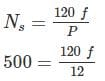F = 50 Hz
For I.M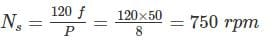Nr = (1 - S) Ns = (1 - .03) × 750
∴ Nr = 727.5 RPM

Test: Electrical Machines- 7 - Question 3

### At a location in India, it is necessary to supply 300 kW of 60 Hz power. The only power source available operate at 50 Hz. It is decide to generate the power by means of a motor-generator set consisting of a synchronous motor driving synchronous generator. How many pole should each of the two machine have in order to convert 50 Hz power to 60-Hz power?

Detailed Solution for Test: Electrical Machines- 7 - Question 3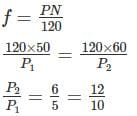∴ A 10-pole synchronous motor must be coupled to a 12-pole synchronous generator to accomplish this frequency conversion.

Test: Electrical Machines- 7 - Question 4

A synchronous generator connected to an Infinite bus is under excited. Considering only the reactive power, from the point of the system the machine act as

Detailed Solution for Test: Electrical Machines- 7 - Question 4

For generator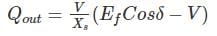When Ef cosδ < V, i.e under excited generator, then reactive power (Qout) is negative i.e supplying leading VARs and therefore operating at leading power factor, thus behaving like a capacitor.

*Answer can only contain numeric values
Test: Electrical Machines- 7 - Question 5

A 30 KVA, 230 V, Y connected, 3 phase salient pale synchronous generator supplies rated load at 0.707 lagging power factor. The reactance per phase are Xd = 2Xq = 4 Ω. Neglect the armature resistance determine the power angle (in degree).

Detailed Solution for Test: Electrical Machines- 7 - Question 5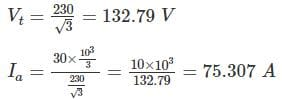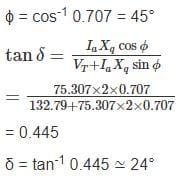Test: Electrical Machines- 7 - Question 6

A 3 phase alternator has generated emf per phase of 300 V with 15% third harmonic and 10% fifth harmonic content. If the alternator is star connected, what is the value of rms line voltage?

Detailed Solution for Test: Electrical Machines- 7 - Question 6

In star connection, the third harmonic component of the three phases cancel out at the line terminals because they are co phased.
∴ the line emf will be consisting of the fundamental and fifth harmonic only
Fundamental component E1 = 300 V
5th harmonic component, E5 = 0.1 × 300 = 30 V
Emf per phase,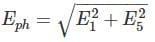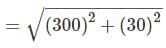= 301.5 V
rms line voltage,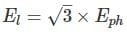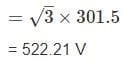*Answer can only contain numeric values
Test: Electrical Machines- 7 - Question 7

A salient pole synchronous generator has Xof 0.90 PU and Xq of 0.6 PU. The armature resistance is negligible and generator delivers rated KVA at unity P.F and rated terminal voltage. What is the value of excitation voltage?

Detailed Solution for Test: Electrical Machines- 7 - Question 7

Generated voltage of salient pole synchronous generator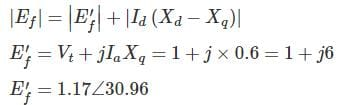Direct component of current
Id = Ia sin 30.96 = 0.5145 PU
∴ |EF| = 1.17 + .5145 × 0.3 = 1.324 PU

*Answer can only contain numeric values
Test: Electrical Machines- 7 - Question 8

A 4 pole 50 Hz star connected alternator has 8 slots/pole/phase, conductor per slot being 8 and flux per pale 0.28 wb. If the winding coil span is 150°, the inductor phase voltage is ________ V.

Detailed Solution for Test: Electrical Machines- 7 - Question 8

Given
Slots/pole/phase m = 8
Conductor/slot = 9
Coil/pole = 24
Slot = 24 × 4 = 96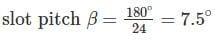Total no. of conductor z = 96 × 8 = 768
Conductor/phase = 256
Number of turn = 128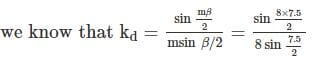= 0.9556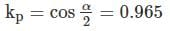Eph = 4.44 × 0.9556 × 0.965 × 0.28 × 50 × 128 = 7337.09 V

Test: Electrical Machines- 7 - Question 9

An alternator has 18 slots/pole and first coil lies in slots 1 and 16. What is the ratio of pitch factor for 3rd harmonic and 5th harmonic

Detailed Solution for Test: Electrical Machines- 7 - Question 9

Coil span is 16 – 1 = 15 slots
Number of empty slots = 18 – 15 = 3 slots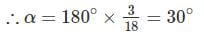Pitch factor for 3rd harmonic,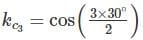= 0.707
Pitch factor for 5th harmonic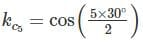= 0.259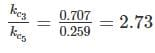*Answer can only contain numeric values
Test: Electrical Machines- 7 - Question 10

A 30 KVA, 230 V, Y connected, 3 phase salient pale synchronous generator supplies rated load at 0.707 lagging power factor. The reactance per phase are Xd = 2Xq = 4 Ω. Neglect the armature resistance determine The percentage voltage regulation__________

Detailed Solution for Test: Electrical Machines- 7 - Question 10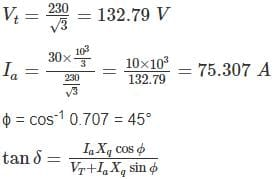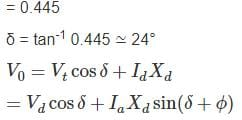= 132.79 × 0.9136 + 75.307 × 4 sin (24 + 45°)
= 121.316 + 281.19
= 402.506
Percentage voltage regulation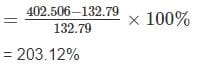## GATE Electrical Engineering (EE) 2023 Mock Test Series

22 docs|274 tests
Information about Test: Electrical Machines- 7 Page
In this test you can find the Exam questions for Test: Electrical Machines- 7 solved & explained in the simplest way possible. Besides giving Questions and answers for Test: Electrical Machines- 7, EduRev gives you an ample number of Online tests for practice

## GATE Electrical Engineering (EE) 2023 Mock Test Series

22 docs|274 tests(Scan QR code)